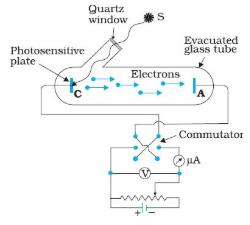Courses

# Test: Dual Nature Of Radiation And Matter (CBSE Level With Solutions)

## 30 Questions MCQ Test Physics Class 12 | Test: Dual Nature Of Radiation And Matter (CBSE Level With Solutions)

Description
This mock test of Test: Dual Nature Of Radiation And Matter (CBSE Level With Solutions) for Class 12 helps you for every Class 12 entrance exam. This contains 30 Multiple Choice Questions for Class 12 Test: Dual Nature Of Radiation And Matter (CBSE Level With Solutions) (mcq) to study with solutions a complete question bank. The solved questions answers in this Test: Dual Nature Of Radiation And Matter (CBSE Level With Solutions) quiz give you a good mix of easy questions and tough questions. Class 12 students definitely take this Test: Dual Nature Of Radiation And Matter (CBSE Level With Solutions) exercise for a better result in the exam. You can find other Test: Dual Nature Of Radiation And Matter (CBSE Level With Solutions) extra questions, long questions & short questions for Class 12 on EduRev as well by searching above.
QUESTION: 1

Solution:
QUESTION: 2

Solution:
QUESTION: 3

### The work function of a photoelectric material is 3.32 eV. The threshold frequency will be equal to

Solution:
QUESTION: 4

Energy of a photon of green light of wavelength 5500m  is (given: h = 6.62 ×10−34Js−1) approximately

Solution:

As we know,
the formula for energy of photon in terms of wavelength is,, E = hc/ λ
where, E = energy of photon
h = Planck's constant =
= 6.63×10-34 Js
c = speed of light
= 3×108 m/s
and lambda = wavelength
so,
E = [6.63×10-34 J. s×3×108 m/s]/5500×10-10m
= [0.36×10-26]/10-8
= 0.36×10-18
= 3.6×10-19 J
Or, 2.26ev

QUESTION: 5

Uniform electric and magnetic fields are produced pointing in the same direction. An electron is projected pointing in the same direction, then

Solution:

Electromagnetic force on electron will be zero V×B=0
Electrostatic force will be Fe​=−E backward, hence electron decelerate and velocity will decrease in magnitude.

QUESTION: 6

In which case is electron emission from a metal not known?

Solution:
QUESTION: 7

Photons can be

Solution:
QUESTION: 8

Given h = 6.6 ×10−34 joule sec, the momentum of each photon in a given radiation is 3.3 ×10−29 kg metre/sec. The frequency of radiation is

Solution:
QUESTION: 9

If the work function of a material is 2eV, then minimum frequency of light required to emit photo-electrons is

Solution:

Φ= hνλ => 2eV= 6.626 x 10-34 x ν

2 x 1.6 x 10-19= 6.626 x 10-34 x ν

On solving we get ν = 4.6 x 1014 Hz.

QUESTION: 10

In Thomson’s method for finding specific charge of positive rays, the electric and magnetic fields are

Solution:
QUESTION: 11

Photoelectric effect is

Solution:
QUESTION: 12

In a photon-particle collision (such as photon-electron collision) the quantity which is not conserved is

Solution:
QUESTION: 13

The specific charge for positive rays is much less than that for cathode rays. This is because

Solution:
QUESTION: 14

Photoelectric effect is possible

Solution:
QUESTION: 15

Davisson Germer experiment proves that

Solution:
QUESTION: 16

If the voltage across the electrodes of a cathode ray tube is 500 volts then energy gained by the electrons is

Solution:
QUESTION: 17

If work function of a metal plate is negligible then the K.E.of the photoelectrons emitted when radiations of 1000 Â are incident on the metal surface is

Solution:
QUESTION: 18

The maximum kinetic energy of photoelectrons emitted from a surface when photons of energy 6 eV fall on it is 4 eV. The stopping potential is

Solution:
QUESTION: 19

In Photoelectric effect

Solution:
QUESTION: 20

A photon is

Solution:
QUESTION: 21

If maximum velocity with which an electron can be emitted from a photo cell is 3.75×108cms−1 then stopping potential is

Solution:
QUESTION: 22

In an experiment of photoelectric emission for incident light of 4000 Â, the stopping potential is 2V. If the wavelength of incident light is made 3000 Â, then stopping potential will be

Solution:
QUESTION: 23

In the above experimental set up for studying photoelectric effect, if keeping the frequency of the incident radiation and the accelerating potential fixed, the intensity of light is varied, thenSolution:
QUESTION: 24

Photo-electric effect can be explained only by assuming that light

Solution:
QUESTION: 25

Wavelength of light incident on a photo cell is 3000 Â, if stopping potential is 2.5 volts, then work function of the cathode of photo cell is

Solution:
QUESTION: 26

in photoelectric effect, the photoelectric current

Solution:
QUESTION: 27

In various experiments on photo electricity the stopping potential for a given frequency of the incident radiation

Solution:
QUESTION: 28

In order to increase the kinetic energy of ejected photoelectrons, there should be an increase in

Solution:
QUESTION: 29

Each photon has the same speed but different

Solution:
QUESTION: 30

Number of ejected photoelectrons increases with increase

Solution: